# h Bessel equation of order p is ty" + ty + (t? - p2 y =...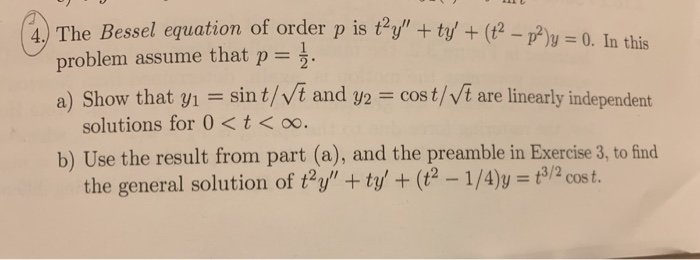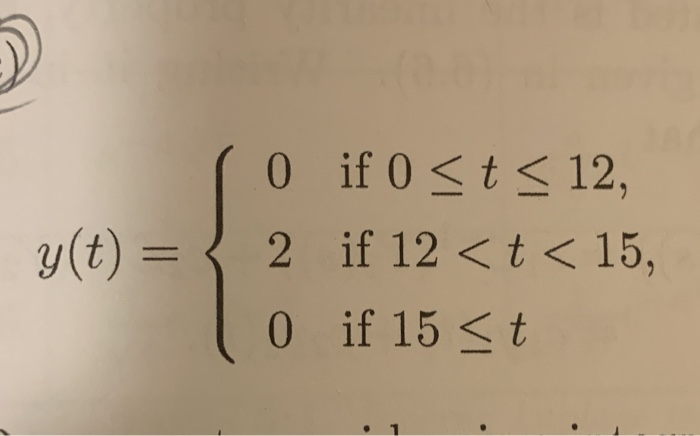h Bessel equation of order p is ty" + ty + (t? - p2 y = 0. In this problem assume that p= 2. a) Show that y1 = sint/Vt and y2 = cost/vt are linearly independent solutions for 0 <t<o. b) Use the result from part (a), and the preamble in Exercise 3, to find the general solution of ty" + ty' + (t2 - 1/4)y = 3/2 cost.
(o if 0 <t < 12, y(t) = { 2 if 12 < t < 15, 0 if 15 <t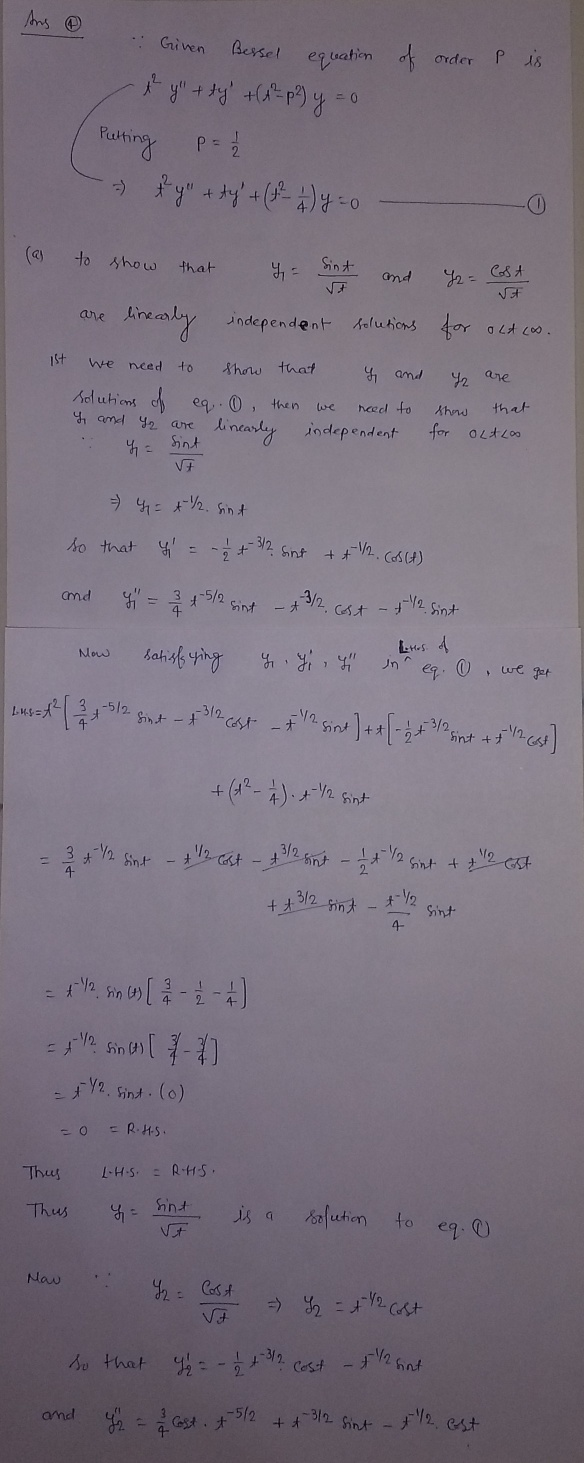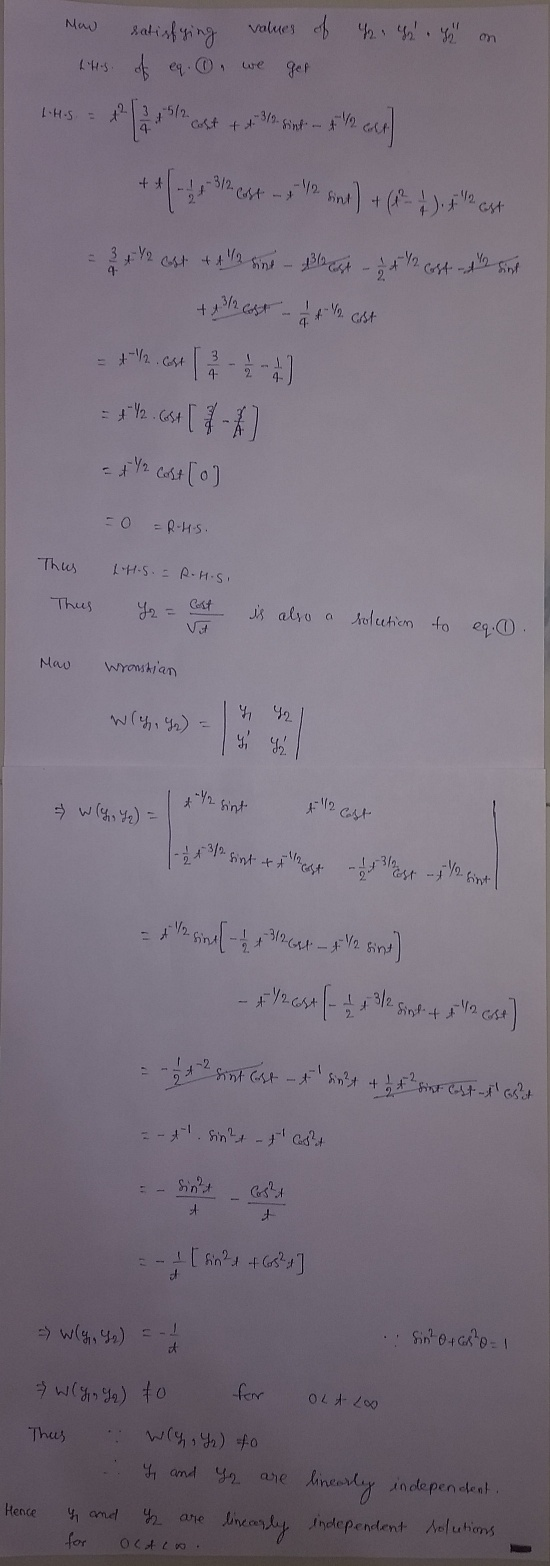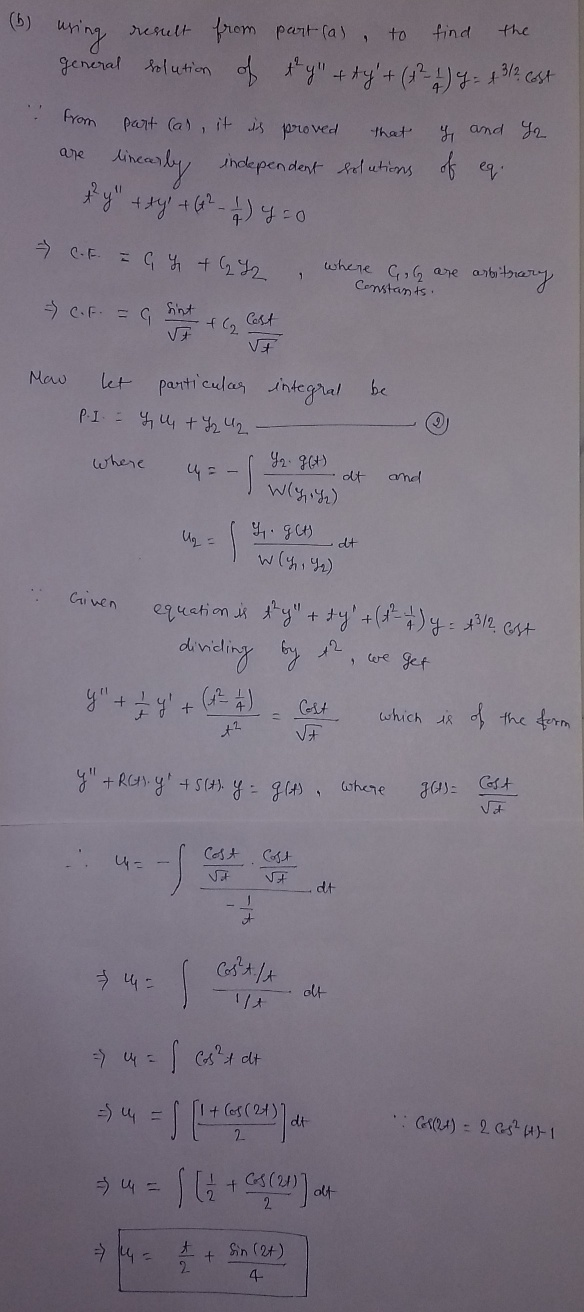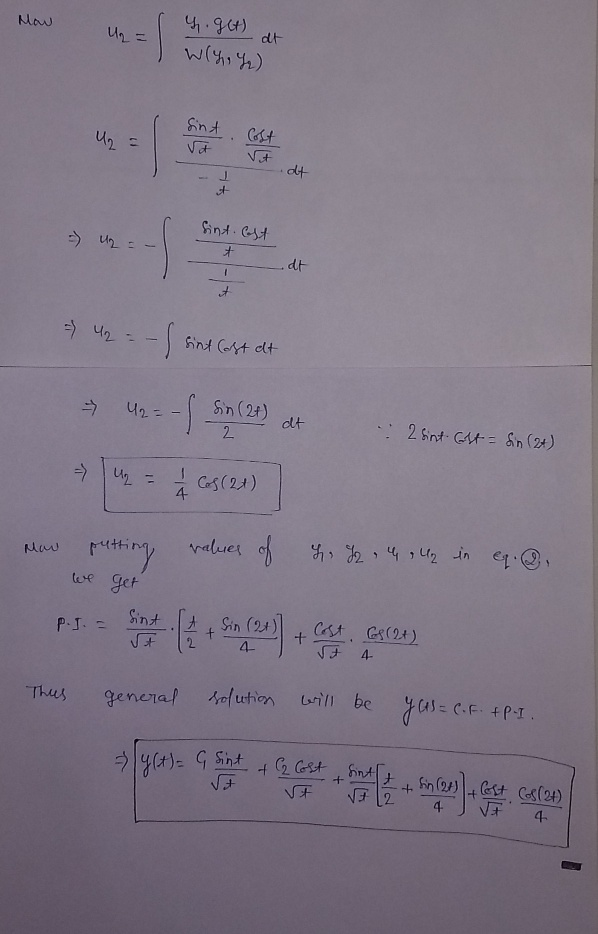#### Earn Coin

Coins can be redeemed for fabulous gifts.

Similar Homework Help Questions
• ### y(0 ' diany ty-o レ 4.(15 pts) Suppose gi(t), va(t) are solutions of (1 + t)y" + (sint)y ey 0. Suppose also that n attains a maximum and y/2 attains a minimum, both at the point t 5. Do y1, /...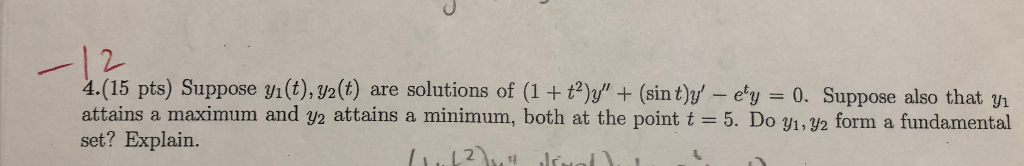y(0 ' diany ty-o レ 4.(15 pts) Suppose gi(t), va(t) are solutions of (1 + t)y" + (sint)y ey 0. Suppose also that n attains a maximum and y/2 attains a minimum, both at the point t 5. Do y1, /2 form a fundamental set? Explain. y(0 ' diany ty-o レ 4.(15 pts) Suppose gi(t), va(t) are solutions of (1 + t)y" + (sint)y ey 0. Suppose also that n attains a maximum and y/2 attains a minimum, both at...

• ### The Bessel equation of order one-half is X .2 dy d.2 + X dy dar +(x2...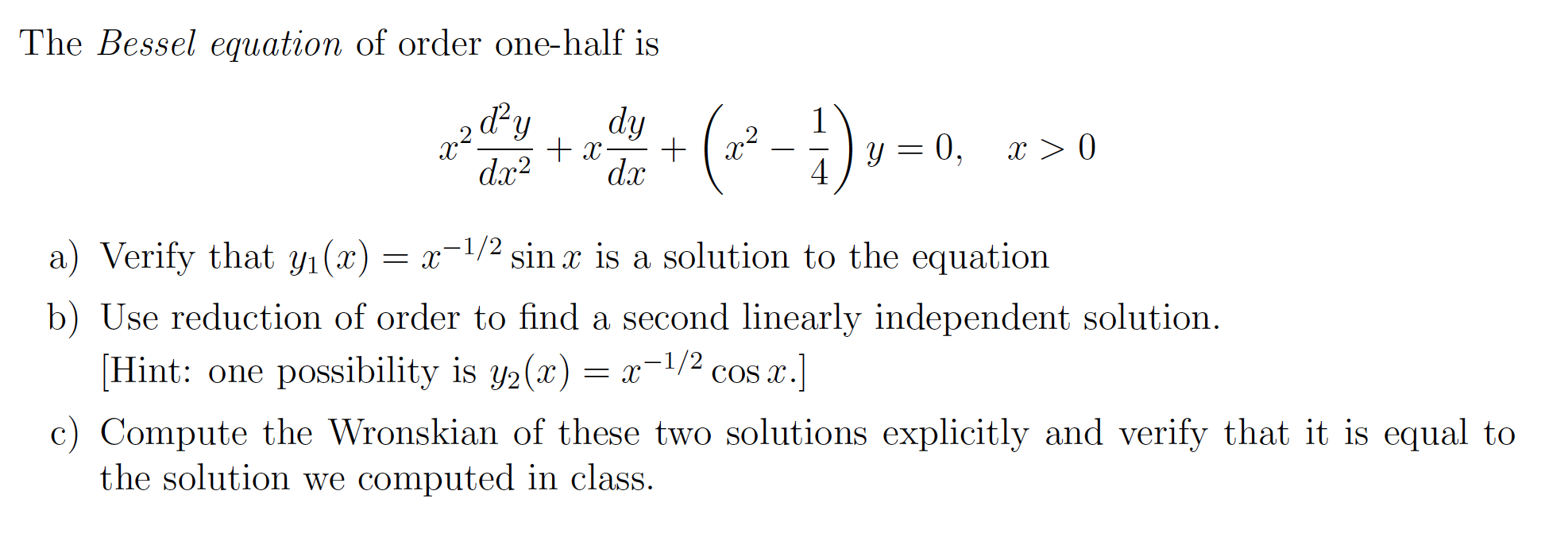The Bessel equation of order one-half is X .2 dy d.2 + X dy dar +(x2 - :) y = 0, X > 0 4 a) Verify that yı(x) = x-1/2 sin x is a solution to the equation b) Use reduction of order to find a second linearly independent solution. (Hint: one possibility is y2(x) = x-1/2 cos x.] c) Compute the Wronskian of these two solutions explicitly and verify that it is equal to the solution we computed...

• ### Use Reduction of Order method to find the second linearly independent solution: t2y``- ty`+y = 0....

Use Reduction of Order method to find the second linearly independent solution: t2y``- ty`+y = 0. y1=t

• ### If y is a known nonvanishing solution of y" p(t)y + q(t)y 0, then a second...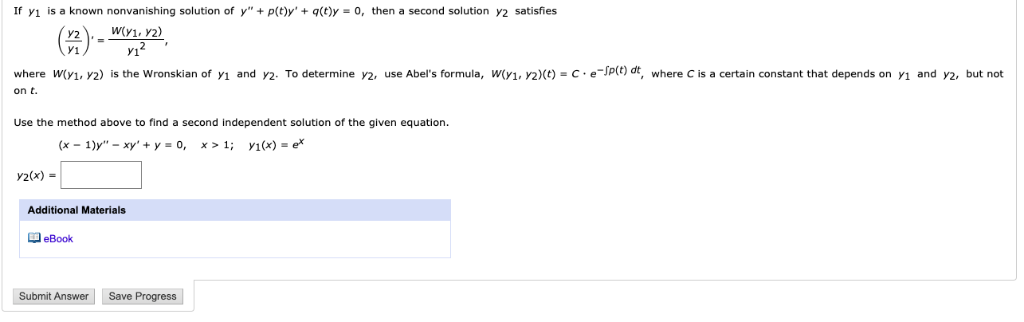If y is a known nonvanishing solution of y" p(t)y + q(t)y 0, then a second solution y2 satisfies 2 У1? where W(y1, y2) is the Wronskian of y1 and y2. To determine y2, use Abel's formula, W(y1, Y2)(t) =C.eJP(t) dt, where C is certain constant that depends on y1 and y2, but not on t. Use the method above o find a second independent solution of the given equation. (х — 1)у" - ху" + у %3D 0, x>...

• ### Two linearly independent solutions of the differential equation y" - 5y' + 6y = 0 are...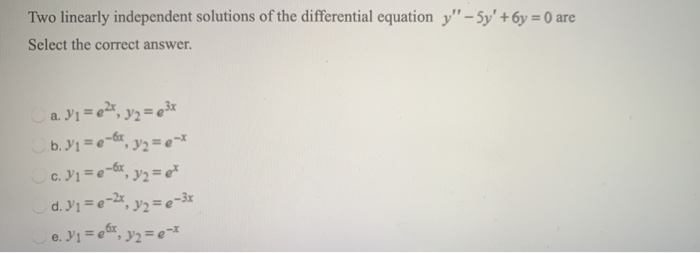Two linearly independent solutions of the differential equation y" - 5y' + 6y = 0 are Select the correct answer. a. Y1 = 62, y2 = 232 b. Y1 = 0 -6x, y2 = e** c. Y1 = e-Gx, y2 = et d. Y1 = 0-2, y2 = 2-3x e. Yi = e6x, y2 = e-*

• ### 1. Consider the polynomial p.(t)=1+t2 and pz(t)=1 – 12. Is {P1, P2} a linearly independent set...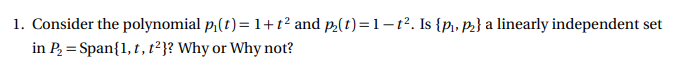1. Consider the polynomial p.(t)=1+t2 and pz(t)=1 – 12. Is {P1, P2} a linearly independent set in P = Span{1,1, 12? Why or Why not?

• ### 2. Let the random variables Y1 and Y, have joint density Ayſy22 - y2) 0<yi <1,...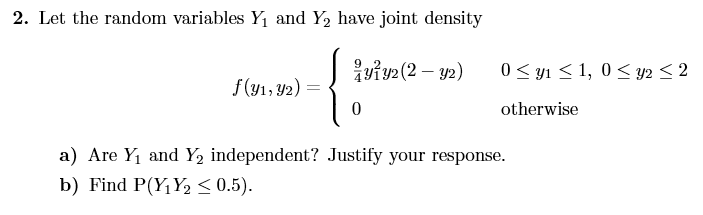2. Let the random variables Y1 and Y, have joint density Ayſy22 - y2) 0<yi <1, 0 < y2 < 2 f(y1, y2) = { otherwise Stom.vn) = { isiml2 –») 05451,05 ms one a independent, amits your respon a) Are Y1 and Y2 independent? Justify your response. b) Find P(Y1Y2 < 0.5). on the

• ### Given the path C: x(t) = (cost, sint, t), 0<t<2n. Let f(t, y, z) = x2...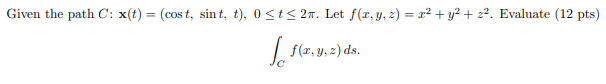Given the path C: x(t) = (cost, sint, t), 0<t<2n. Let f(t, y, z) = x2 + y2 + 22. Evaluate (12 pts) f(,y,z)ds.

• ### Three linearly independent solutions of the differential equation y'"' - y" - 6y' = 0 are...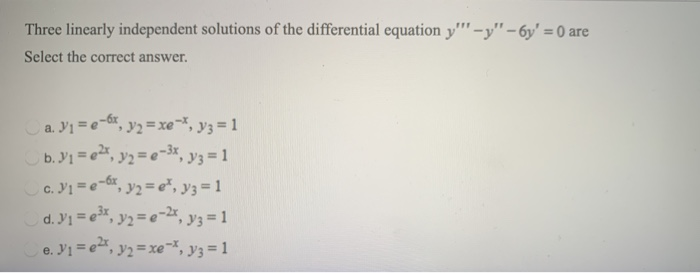Three linearly independent solutions of the differential equation y'"' - y" - 6y' = 0 are Select the correct answer. a. V1 =e-6s, y2 =xe-1, V3 =1 b. Y1 = 224, y2 = 2-3x, y3 = 1 c. Y1 = 2-6x, y2 = e", y3 = 1 d. Y1 = e3x, y2 = 2-2*, y3 = 1 e. Vi=e , y2=xe-1, V3=1

• ### Two linearly independent solutions of the differential equation y" + 4y' + 5y = 0 are...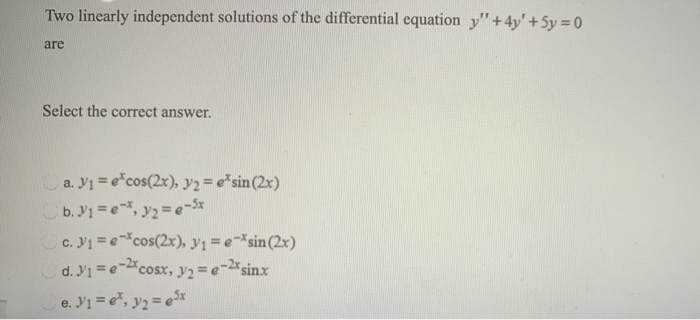Two linearly independent solutions of the differential equation y" + 4y' + 5y = 0 are Select the correct answer. a. Y1 = e-cos(2x), y2 = eʼsin (2x) b. Y1 = e-*, y2 = e-S* c. Yi= e-*cos(2x), y1=e-* sin(2x) d. Y1 = e-2xcosx, x, y2 = e–2*sinx e. Y1 = e', y2 = 5x